• This is an assessment test.
• These tests focus on the basics of Maths and are meant to indicate your preparation level for the subject.
• Kindly take the tests in this series with a pre-defined schedule.

## Basic Maths: Test 1

Congratulations - you have completed Basic Maths: Test 1. You scored %%SCORE%% out of %%TOTAL%%. You correct answer percentage: %%PERCENTAGE%% . Your performance has been rated as %%RATING%%
 Question 1
Simplify: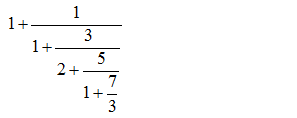A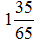B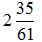C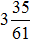D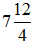Question 1 Explanation:
We do this question step by step from the lower part of the question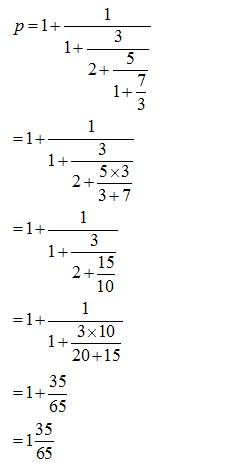Question 2
Simplify: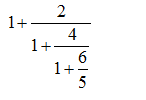A 7/4 B 312/7 C 53/31 D 80/7
Question 2 Explanation:
We do this question step by step from the lower part of the question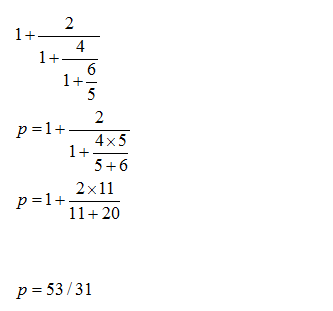Question 3
The value of is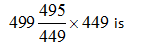A 224546 B 234546 C 223546 D 264546
Question 3 Explanation:
The Given Expression is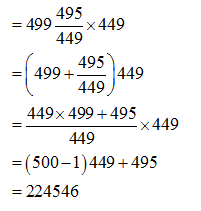Question 4
Find the value of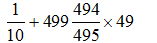A 22050 B 24500 C 22050 D 2.16297e+07
Question 4 Explanation: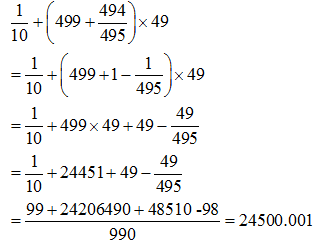Question 5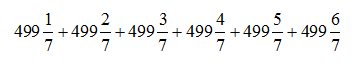is simplified to
 A 5997 B 5979 C 5994 D 2997
Question 5 Explanation: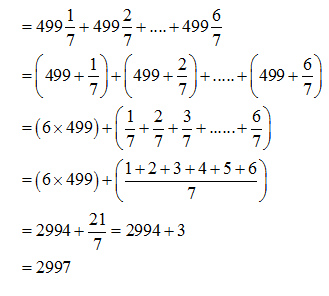Once you are finished, click the button below. Any items you have not completed will be marked incorrect.
There are 5 questions to complete.
 ← List →
Shaded items are complete.
 1 2 3 4 5 End